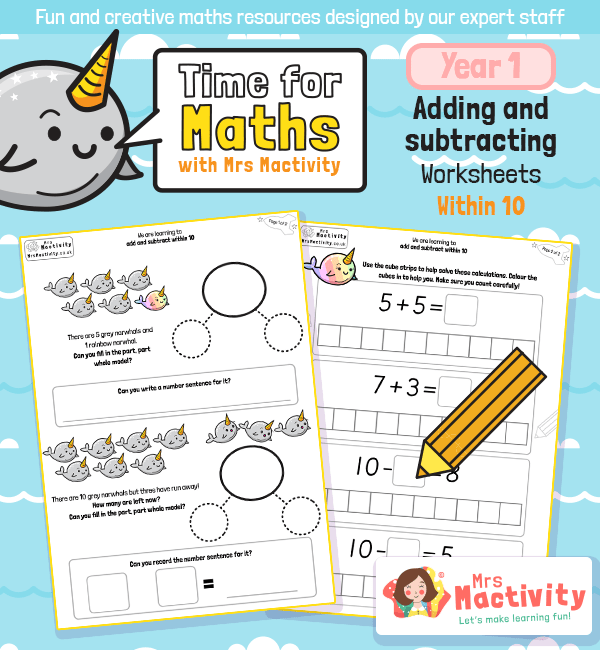# Year 1 Adding and Subtracting Within 10 WorksheetsHelp children get to grips with maths mastery with this Year 1 Adding and Subtracting Within 10 Worksheets.  This covers the year 1 maths objective:

• represent and use number bonds and related subtraction facts within 20
• add and subtract one-digit and two-digit numbers to 20, including zero
• solve one-step problems that involve addition and subtraction, using concrete objects and pictorial representations, and missing number problems such as 7 = – 9.

Explore our year 1 maths resources.

Explore our maths scheme.

Explore our addition and subtraction resources.

## Recently Viewed